In our article LM317 Voltage Regulator we introduced the LM317 which can provide a regulated output voltage from 1.2-37V from an input voltage of 3-40V, with the output voltage set simply with a couple of resistors.

In this article we will show how this IC can be used to make an adjustable power supply by replacing one of the two voltage setting resistors with a potentiometer (variable resistor).

### Adjustable Power Supply with LM317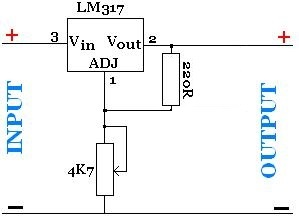Pictured above is the circuit diagram from an adjustable power supply. The two resistors used to set the output voltage from the LM317 are called R1 and R2. In this adjustable supply, R1 is fixed at 220 Ohms, and R2 is a 4k7 potentiometer which means that R2 has a range of 0-4,700 Ohms which can be selected by rotating the potentiometer.

Using our LM317 Voltage Calculator it can be seen that if R1 = 220 and R2 = 0 the output voltage is 1.26V, and if R1 = 220 and R2 = 4700 the output voltage is 28V. These figures alone do not tell the full story though as the output voltage will always be 2-3 Volts lower than the input voltage. Therefore for example, if the input voltage is 15V the highest output voltage possible will be around 12-13V.

### High Current Power Supply with LM317T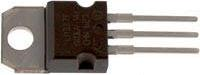The LM317T with correct heatsinking (see our article LM317T Heatsinking) can output a maximum constant current of around 1.5 Amps. If you need more current, then the circuit has to be extended to incorporate one or more power transistors to take the majority of the load away from the LM317T.

In our article High Current Voltage Regulator we showed one way in which an LM317 could be coupled with a transistor to supply high currents. Replacing R2 in that circuit with a potentiometer would make a high current adjustable power supply.

An alternative high current adjustable power supply ciircuit design is shown below: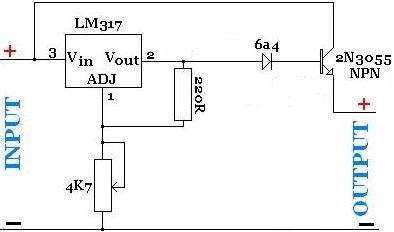Here the adjustable power supply circuit has been modified with the addition of a diode (6a4) and anNPN power transistor (2N3055). Different types of 2N3055 (pictured below) are available with different maximum current carrying ratings.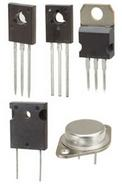For a low to medium sized power supply, it is usually possible to put together the power supply with just the one NPN power transistor with heatsinking. If however a high current power supply is required, this circuit design can be further extended simply by adding more transistors in parallel with the first. The more transistors there are, the higher the current that can be supplied.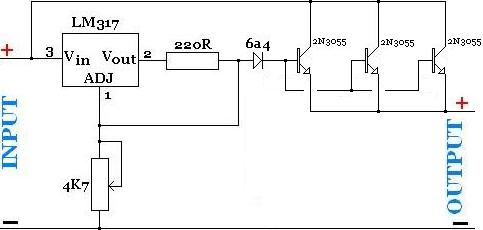The circuit diagram above shows an adjustable power supply with three NPN power transistors, but even more transistors can be added if required for more current.